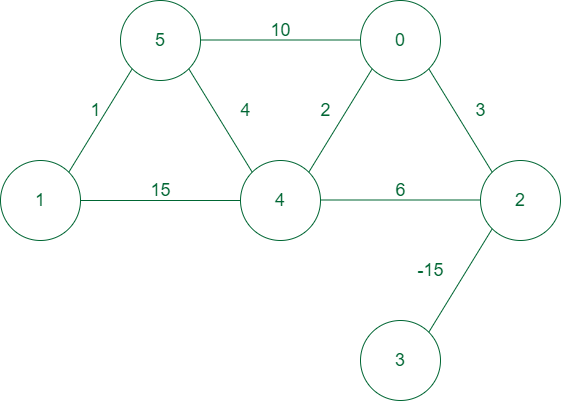Open in App
Not now

• Difficulty Level : Hard
• Last Updated : 09 Feb, 2023

A weighted graph is a special type of graph in which the edges are assigned some weights which represent cost, distance and many other relative measuring units.Example of a weighted graph

Applications of Weighted Graph:

• 2D matrix games: In 2d matrix games can be used to find the optimal path for maximum sum along starting to ending points and many variations of it can be found online.
• Spanning trees: Weighted graphs are used to find the minimum spanning tree from graph which depicts the minimal cost to traverse all nodes in the graph.
• Constraints graphs: Graphs are often used to represent constraints among items. Used in scheduling, product design, asset allocation, circuit design, and artificial intelligence.
• Dependency graphs: Directed weighted graphs can be used to represent dependencies or precedence order among items. Priority will be assigned to provide a flow in which we will solve the problem or traverse the graph from highest priority to lowest priority. Such graphs are often used in large projects in laying out what components rely on other components and are used to minimize the total time or cost to completion while abiding by the dependencies.
• Compilers: Weighted graphs are used extensively in compilers. They can be used for type inference, for so-called data flow analysis, and many other purposes such as query optimization in database languages.
• Artificial Intelligence: Weighted graphs are used in artificial intelligence for decision-making processes, such as in game trees for determining the best move in a game.
• Image Processing: Weighted graphs are used in image processing for segmentation, where the weight of the edges represents the similarity between two pixels.
• Natural Language Processing: Weighted graphs are used in natural language processing for text classification, where the weight of the edges represents the similarity between two words.

Real-Time Applications of Weighted Graph:

• Transportation networks: Using weighted graphs, we can figure things out like the path that takes the least time, or the path with the least overall distance. This is a simplification of how weighted graphs can be used for more complex things like a GPS system. Graphs are used to study traffic patterns, traffic light timings and much more by many big tech companies such as OLA, UBER, RAPIDO, etc. Graph networks are used by many map programs such as Google Maps, Bing Maps, etc.
• Document link graphs:  Link weighted graphs are used to analyze relevance of web pages, the best sources of information, and good link sites by taking the count of the number of views as weights in the graph.
• Epidemiology: Weighted graphs can be used to find the maximum distance transmission from an infectious to a healthy person.
• Graphs in quantum field theory: Vertices represent states of a quantum system and the edges represent transitions between them. The graphs can be used to analyze path integrals and summing these up generates a quantum amplitude. Research to find maximum frequency along a path can be done using weighted graphs.
• Social network graphs: We can find which all users are connected in a network both directly(direct connection) and indirectly(indirect connection). But now weighted graphs are also used in social media for many purposes, for example, In recent times Instagram is using features like close friends which is not the same as all friends these features are being implemented using weighted graphs.
• Network packet traffic graphs: Network packet traffic graphs are used for analyzing network security, studying the spread of worms, and tracking criminal or non-criminal activity.

• It can be used to represent complex social networks, circuit boards, and many more types of complex real-life applications which cannot be implemented by any other data structure.
• It can be used to find the shortest path between any two nodes.
• The spanning tree concept helps users to find a path that visits all nodes of the graph with minimum cost.
• Weighted graphs can be used to solve optimization problems, such as finding the shortest path or maximum flow between two nodes.
• Weighted graphs can be used to support decision-making processes, such as in AI and financial planning.